# semilogy

Semilog plot (y-axis has log scale)

•## Syntax

``semilogy(X,Y)``
``semilogy(X,Y,LineSpec)``
``semilogy(X1,Y1,...,Xn,Yn)``
``semilogy(X1,Y1,LineSpec1,...,Xn,Yn,LineSpecn)``
``semilogy(Y)``
``semilogy(Y,LineSpec)``
``semilogy(___,Name,Value)``
``semilogy(ax,___)``
``lineobj = semilogy(___)``

## Description

example

````semilogy(X,Y)` plots x- and y-coordinates using a linear scale on the x-axis and a base-10 logarithmic scale on the y-axis. To plot a set of coordinates connected by line segments, specify `X` and `Y` as vectors of the same length.To plot multiple sets of coordinates on the same set of axes, specify at least one of `X` or `Y` as a matrix. ```

example

````semilogy(X,Y,LineSpec)` creates the plot using the specified line style, marker, and color.```

example

````semilogy(X1,Y1,...,Xn,Yn)` plots multiple pairs of x- and y-coordinates on the same set of axes. Use this syntax as an alternative to specifying coordinates as matrices.```

example

````semilogy(X1,Y1,LineSpec1,...,Xn,Yn,LineSpecn)` assigns specific line styles, markers, and colors to each x-y pair. You can specify `LineSpec` for some x-y pairs and omit it for others. For example, `semilogy(X1,Y1,'o',X2,Y2)` specifies markers for the first x-y pair but not the for the second pair.```

example

````semilogy(Y)` plots `Y` against an implicit set of x-coordinates. If `Y` is a vector, the x-coordinates range from 1 to `length(Y)`.If `Y` is a matrix, the plot contains one line for each column in `Y`. The x-coordinates range from 1 to the number of rows in `Y`. If `Y` contains complex numbers, `semilogy` plots the imaginary part of `Y` versus the real part of `Y`. However, if you specify both `X` and `Y`, MATLAB® ignores the imaginary part.```
````semilogy(Y,LineSpec)` specifies line style, marker, and color.```

example

````semilogy(___,Name,Value)` specifies `Line` properties using one or more `Name,Value` pair arguments. The properties apply to all the plotted lines. Specify the `Name,Value` pairs after all the arguments in any of the previous syntaxes. For a list of properties, see Line Properties.```

example

````semilogy(ax,___)` displays the plot in the target axes. Specify the axes as the first argument in any of the previous syntaxes.```

example

````lineobj = semilogy(___)` returns a `Line` object or an array of `Line` objects. Use `lineobj` to modify properties of the plot after creating it. For a list of properties, see Line Properties.```

## Examples

collapse all

Create a vector of x-coordinates and a vector of y-coordinates. Create a log-linear plot of `x` and `y`, and call the `grid` function to show the grid lines.

```x = 1:100; y = x.^2; semilogy(x,y) grid on```Create a vector of x-coordinates and two vectors of y-coordinates. Plot two lines by passing comma-separated x-y pairs to `semilogy`.

```x = 1:100; y1 = x.^2; y2 = x.^3; semilogy(x,y1,x,y2) grid on```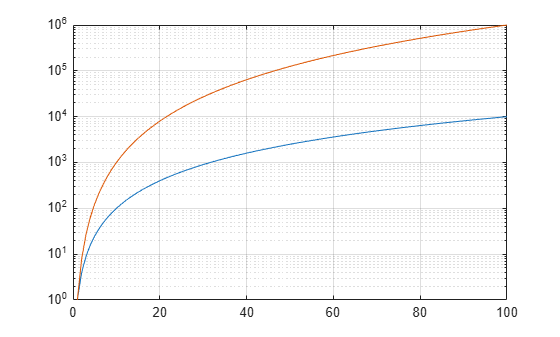Define vector `x` as the installments on a 20 year loan. Define vector `y` as the cumulative cost of a \$1000 loan with an interest rate of 8%. Plot the cumulative cost at each installment.

```P = 1000; npayments = 240; rate = 0.08/12; mpayment = P*(rate*(1+rate)^npayments)/(((1+rate)^npayments) - 1); x = 1:240; y = x * mpayment; semilogy(x,y); grid on```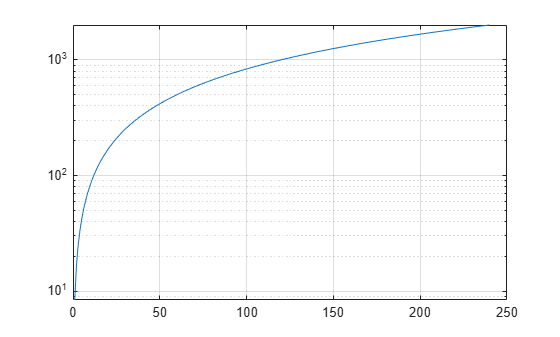Change the y-axis tick values and tick labels by calling the `yticks` and `yticklabels` functions. Then create x- and y-axis labels by calling the `xlabel` and `ylabel` functions.

```yticks([10 50 100 500 1000]) yticklabels({'\$10','\$50','\$100','\$500','\$1000'}) xlabel ('Installment') ylabel('Cumulate Cost')```Create a set of x- and y-coordinates and plot them in a log-linear plot. Specify the line style as `'o'` to display circular markers without connecting lines. Specify the marker fill color as the RGB triplet `[0 0.447 0.741]`, which corresponds to a dark shade of blue.

```x = linspace(1,1000,15); y = (1./x) * 10000; semilogy(x,y,'o','MarkerFaceColor',[0 0.447 0.741]) grid on```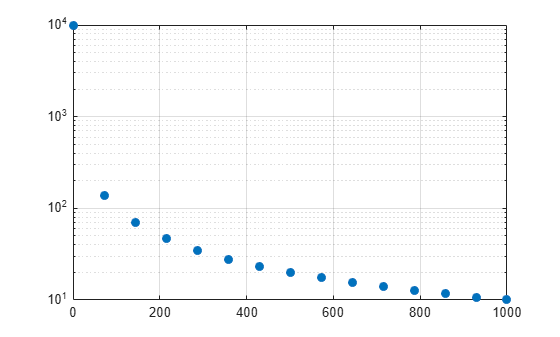Create two sets of x- and y-coordinates and display them in a log-linear plot. Specify a dashed line for the first set of coordinates. Then display a legend in the upper left corner of the plot by calling the `legend` function and specifying the location as `'northwest'`.

```x = 1:100; y1 = x.^2; y2 = x.^3; semilogy(x,y1,'--',x,y2) legend('x^2','x^3','Location','northwest')```When you specify only one coordinate vector, `semilogy` plots those coordinates against the values `1:length(y)`. For example, define `y` as a vector of 5 values. Create a log-linear plot of y.

```y = [0.1 0.2 1 10 1000]; semilogy(y) grid on```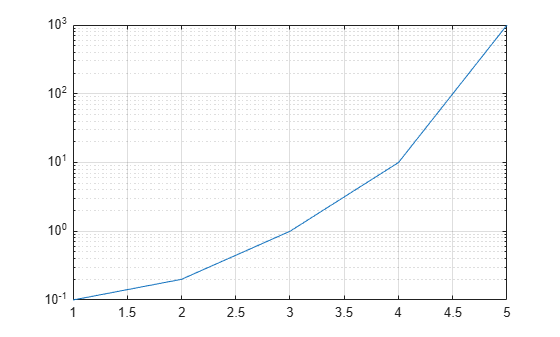If you specify `y` as a matrix, the columns of y are plotted against the values `1:size(y,1)`. For example, define `y` as a 5-by-3 matrix and pass it to the `semilogy` function. The resulting plot contains 3 lines, each of which has x-coordinates that range from `1` to `5`.

```y = [ 0.1 1 10 0.2 2 20 1.0 10 100 10 100 1000 1000 10000 100000]; semilogy(y) grid on```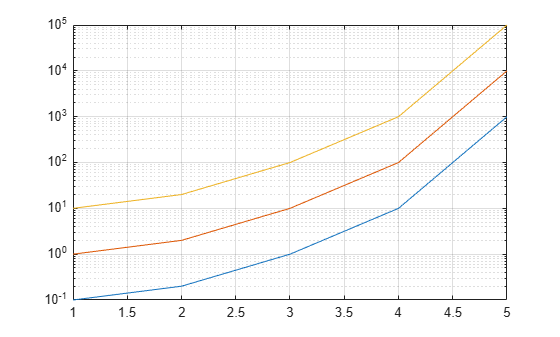Create a tiled chart layout in the `'flow'` tile arrangement, so that the axes fill the available space in the layout. Next, call the `nexttile` function to create an axes object and return it as `ax1`. Then display a log-linear plot by passing `ax1` to the `semilogy` function.

```tiledlayout('flow') ax1 = nexttile; x = 1:100; y1 = x.^2; semilogy(ax1,x,y1)```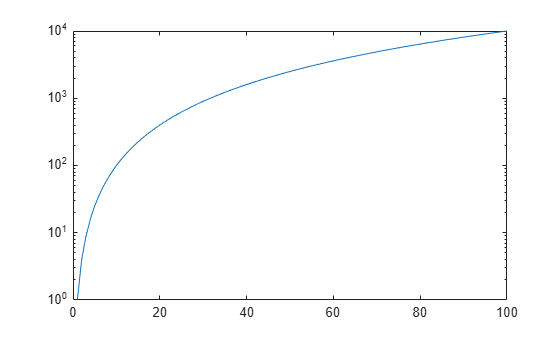Repeat the process to create a second log-linear plot.

```ax2 = nexttile; y2 = 1./x; semilogy(ax2,x,y2)```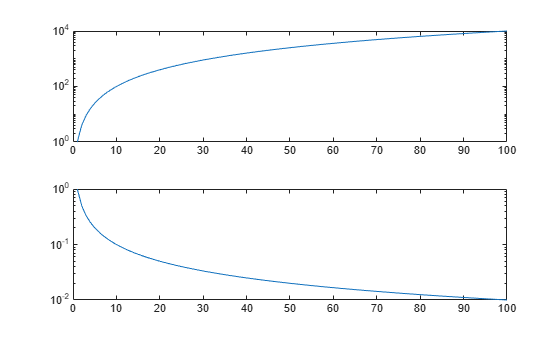Create a log-linear plot containing two lines, and return the line objects in the variable `slg`.

```x = 1:100; y1 = x.^2; y2 = x.^3; slg = semilogy(x,y1,x,y2);```Change the width of the first line to `3`, and change the color of the second line to purple.

```slg(1).LineWidth = 3; slg(2).Color = [0.4 0 1];```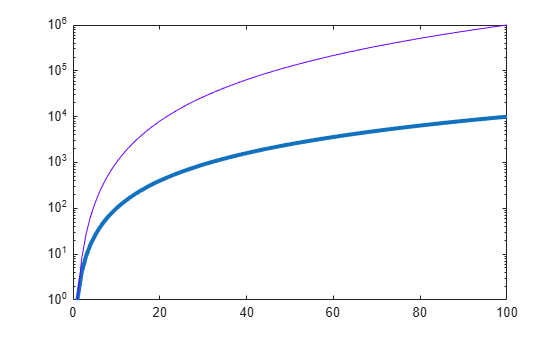Insert `NaN` values wherever there are discontinuities in your data. The `semilogy` function displays gaps at those locations.

Create a pair of x- and y-coordinate vectors. Replace the twentieth y-coordinate with a `NaN` value. Then create a log-linear plot of `x` and `y`.

```x = 1:50; y = x.^2; y(20) = NaN; semilogy(x,y)```## Input Arguments

collapse all

Linear scale coordinates, specified as a scalar, vector, or matrix. The size and shape of `X` depends on the shape of your data and the type of plot you want to create. This table describes the most common situations.

Type of PlotHow to Specify Coordinates
Single point

Specify `X` and `Y` as scalars and include a marker. For example:

`semilogy(1,2,'o')`

One set of points

Specify `X` and `Y` as any combination of row or column vectors of the same length. For example:

`semilogy([1 2 3],[4; 5; 6])`

Multiple sets of points
(using vectors)

Specify consecutive pairs of `X` and `Y` vectors. For example:

`semilogy([1 2 3],[4 5 6],[1 2 3],[7 8 9])`

Multiple sets of points
(using matrices)

If all the sets share the same x- or y-coordinates, specify the shared coordinates as a vector and the other coordinates as a matrix. The length of the vector must match one of the dimensions of the matrix. For example:

`semilogy([1 2 3],[4 5 6; 7 8 9])`
If the matrix is square, `semilogy` plots one line for each column in the matrix.

Alternatively, specify `X` and `Y` as matrices of equal size. In this case, `semilogy` plots each column of `Y` against the corresponding column of `X`. For example:

`semilogy([1 2 3; 4 5 6],[7 8 9; 10 11 12])`

Data Types: `single` | `double` | `int8` | `int16` | `int32` | `int64` | `uint8` | `uint16` | `uint32` | `uint64` | `categorical` | `datetime` | `duration`

Log scale coordinates, specified as a scalar, vector, or matrix. The size and shape of `Y` depends on the shape of your data and the type of plot you want to create. This table describes the most common situations.

Type of PlotHow to Specify Coordinates
Single point

Specify `X` and `Y` as scalars and include a marker. For example:

`semilogy(1,2,'o')`

One set of points

Specify `X` and `Y` as any combination of row or column vectors of the same length. For example:

`semilogy([1 2 3],[4; 5; 6])`

Multiple sets of points
(using vectors)

Specify consecutive pairs of `X` and `Y` vectors. For example:

`semilogy([1 2 3],[4 5 6],[1 2 3],[7 8 9])`

Multiple sets of points
(using matrices)

If all the sets share the same x- or y-coordinates, specify the shared coordinates as a vector and the other coordinates as a matrix. The length of the vector must match one of the dimensions of the matrix. For example:

`semilogy([1 2 3],[4 5 6; 7 8 9])`
If the matrix is square, `semilogy` plots one line for each column in the matrix.

Alternatively, specify `X` and `Y` as matrices of equal size. In this case, `semilogy` plots each column of `Y` against the corresponding column of `X`. For example:

`semilogy([1 2 3; 4 5 6],[7 8 9; 10 11 12])`

`semilogy` might exclude coordinates in some cases:

• If the log scale coordinates include positive and negative values, only the positive values are displayed.

• If the log scale coordinates are all negative, all of the values are displayed on a log scale with the appropriate sign.

• Log scale values of zero are not displayed.

Data Types: `single` | `double` | `int8` | `int16` | `int32` | `int64` | `uint8` | `uint16` | `uint32` | `uint64`

Line style, marker, and color, specified as a character vector or string containing symbols. The symbols can appear in any order. You do not need to specify all three characteristics (line style, marker, and color). For example, if you omit the line style and specify the marker, then the plot shows only the marker and no line.

Example: `'--or'` is a red dashed line with circle markers

Line StyleDescriptionResulting Line
`'-'`Solid line`'--'`Dashed line`':'`Dotted line`'-.'`Dash-dotted lineMarkerDescriptionResulting Marker
`'o'`Circle`'+'`Plus sign`'*'`Asterisk`'.'`Point`'x'`Cross`'_'`Horizontal line`'|'`Vertical line`'s'`Square`'d'`Diamond`'^'`Upward-pointing triangle`'v'`Downward-pointing triangle`'>'`Right-pointing triangle`'<'`Left-pointing triangle`'p'`Pentagram`'h'`HexagramColor NameShort NameRGB TripletAppearance
`'red'``'r'``[1 0 0]``'green'``'g'``[0 1 0]``'blue'``'b'``[0 0 1]``'cyan'` `'c'``[0 1 1]``'magenta'``'m'``[1 0 1]``'yellow'``'y'``[1 1 0]``'black'``'k'``[0 0 0]``'white'``'w'``[1 1 1]`Target axes, specified as an `Axes` object. If you do not specify the axes and if the current axes is Cartesian, then `semilogy` uses the current axes.

### Name-Value Arguments

Specify optional comma-separated pairs of `Name,Value` arguments. `Name` is the argument name and `Value` is the corresponding value. `Name` must appear inside quotes. You can specify several name and value pair arguments in any order as `Name1,Value1,...,NameN,ValueN`.

Example: `semilogy([1 2],[3 4],'Color','red')` specifies a red line for the plot.

Note

The properties listed here are only a subset. For a complete list, see Line Properties.

Color, specified as an RGB triplet, a hexadecimal color code, a color name, or a short name. The color you specify sets the line color. It also sets the marker edge color when the `MarkerEdgeColor` property is set to `'auto'`.

For a custom color, specify an RGB triplet or a hexadecimal color code.

• An RGB triplet is a three-element row vector whose elements specify the intensities of the red, green, and blue components of the color. The intensities must be in the range `[0,1]`; for example, ```[0.4 0.6 0.7]```.

• A hexadecimal color code is a character vector or a string scalar that starts with a hash symbol (`#`) followed by three or six hexadecimal digits, which can range from `0` to `F`. The values are not case sensitive. Thus, the color codes `'#FF8800'`, `'#ff8800'`, `'#F80'`, and `'#f80'` are equivalent.

Alternatively, you can specify some common colors by name. This table lists the named color options, the equivalent RGB triplets, and hexadecimal color codes.

Color NameShort NameRGB TripletHexadecimal Color CodeAppearance
`'red'``'r'``[1 0 0]``'#FF0000'``'green'``'g'``[0 1 0]``'#00FF00'``'blue'``'b'``[0 0 1]``'#0000FF'``'cyan'` `'c'``[0 1 1]``'#00FFFF'``'magenta'``'m'``[1 0 1]``'#FF00FF'``'yellow'``'y'``[1 1 0]``'#FFFF00'``'black'``'k'``[0 0 0]``'#000000'``'white'``'w'``[1 1 1]``'#FFFFFF'``'none'`Not applicableNot applicableNot applicableNo color

Here are the RGB triplets and hexadecimal color codes for the default colors MATLAB uses in many types of plots.

`[0 0.4470 0.7410]``'#0072BD'``[0.8500 0.3250 0.0980]``'#D95319'``[0.9290 0.6940 0.1250]``'#EDB120'``[0.4940 0.1840 0.5560]``'#7E2F8E'``[0.4660 0.6740 0.1880]``'#77AC30'``[0.3010 0.7450 0.9330]``'#4DBEEE'``[0.6350 0.0780 0.1840]``'#A2142F'`Line width, specified as a positive value in points, where 1 point = 1/72 of an inch. If the line has markers, then the line width also affects the marker edges.

The line width cannot be thinner than the width of a pixel. If you set the line width to a value that is less than the width of a pixel on your system, the line displays as one pixel wide.

Marker size, specified as a positive value in points, where 1 point = 1/72 of an inch.

Marker outline color, specified as `'auto'`, an RGB triplet, a hexadecimal color code, a color name, or a short name. The default value of `'auto'` uses the same color as the `Color` property.

For a custom color, specify an RGB triplet or a hexadecimal color code.

• An RGB triplet is a three-element row vector whose elements specify the intensities of the red, green, and blue components of the color. The intensities must be in the range `[0,1]`; for example, ```[0.4 0.6 0.7]```.

• A hexadecimal color code is a character vector or a string scalar that starts with a hash symbol (`#`) followed by three or six hexadecimal digits, which can range from `0` to `F`. The values are not case sensitive. Thus, the color codes `'#FF8800'`, `'#ff8800'`, `'#F80'`, and `'#f80'` are equivalent.

Alternatively, you can specify some common colors by name. This table lists the named color options, the equivalent RGB triplets, and hexadecimal color codes.

Color NameShort NameRGB TripletHexadecimal Color CodeAppearance
`'red'``'r'``[1 0 0]``'#FF0000'``'green'``'g'``[0 1 0]``'#00FF00'``'blue'``'b'``[0 0 1]``'#0000FF'``'cyan'` `'c'``[0 1 1]``'#00FFFF'``'magenta'``'m'``[1 0 1]``'#FF00FF'``'yellow'``'y'``[1 1 0]``'#FFFF00'``'black'``'k'``[0 0 0]``'#000000'``'white'``'w'``[1 1 1]``'#FFFFFF'``'none'`Not applicableNot applicableNot applicableNo color

Here are the RGB triplets and hexadecimal color codes for the default colors MATLAB uses in many types of plots.

`[0 0.4470 0.7410]``'#0072BD'``[0.8500 0.3250 0.0980]``'#D95319'``[0.9290 0.6940 0.1250]``'#EDB120'``[0.4940 0.1840 0.5560]``'#7E2F8E'``[0.4660 0.6740 0.1880]``'#77AC30'``[0.3010 0.7450 0.9330]``'#4DBEEE'``[0.6350 0.0780 0.1840]``'#A2142F'`Marker fill color, specified as `'auto'`, an RGB triplet, a hexadecimal color code, a color name, or a short name. The `'auto'` option uses the same color as the `Color` property of the parent axes. If you specify `'auto'` and the axes plot box is invisible, the marker fill color is the color of the figure.

For a custom color, specify an RGB triplet or a hexadecimal color code.

• An RGB triplet is a three-element row vector whose elements specify the intensities of the red, green, and blue components of the color. The intensities must be in the range `[0,1]`; for example, ```[0.4 0.6 0.7]```.

• A hexadecimal color code is a character vector or a string scalar that starts with a hash symbol (`#`) followed by three or six hexadecimal digits, which can range from `0` to `F`. The values are not case sensitive. Thus, the color codes `'#FF8800'`, `'#ff8800'`, `'#F80'`, and `'#f80'` are equivalent.

Alternatively, you can specify some common colors by name. This table lists the named color options, the equivalent RGB triplets, and hexadecimal color codes.

Color NameShort NameRGB TripletHexadecimal Color CodeAppearance
`'red'``'r'``[1 0 0]``'#FF0000'``'green'``'g'``[0 1 0]``'#00FF00'``'blue'``'b'``[0 0 1]``'#0000FF'``'cyan'` `'c'``[0 1 1]``'#00FFFF'``'magenta'``'m'``[1 0 1]``'#FF00FF'``'yellow'``'y'``[1 1 0]``'#FFFF00'``'black'``'k'``[0 0 0]``'#000000'``'white'``'w'``[1 1 1]``'#FFFFFF'``'none'`Not applicableNot applicableNot applicableNo color

Here are the RGB triplets and hexadecimal color codes for the default colors MATLAB uses in many types of plots.

`[0 0.4470 0.7410]``'#0072BD'``[0.8500 0.3250 0.0980]``'#D95319'``[0.9290 0.6940 0.1250]``'#EDB120'``[0.4940 0.1840 0.5560]``'#7E2F8E'``[0.4660 0.6740 0.1880]``'#77AC30'``[0.3010 0.7450 0.9330]``'#4DBEEE'``[0.6350 0.0780 0.1840]``'#A2142F'`## Tips

• The `semilogy` function uses colors and line styles based on the `ColorOrder` and `LineStyleOrder` properties of the axes. `semilogy` cycles through the colors with the first line style. Then, it cycles through the colors again with each additional line style.

You can change the colors and the line styles after plotting by setting the `ColorOrder` or `LineStyleOrder` properties on the axes. You can also call the `colororder` function to change the color order for all the axes in the figure.

## Algorithms

The `semilogy` function plots y-coordinates on a log scale by setting the `YScale` property of the axes to `'log'`. However, if the axes `hold` state is `'on'` before you call `semilogy`, the property does not change, and the y-coordinates might display on a linear scale.

## Extended Capabilities

### Topics

Introduced before R2006a